﻿ 基于燃油计量阀的高压油泵动态特性仿真分析
 舰船科学技术2017, Vol. 39Issue (10): 97-102PDF

1. 海军工程大学 动力工程学院，湖北 武汉 430033;
2. 中国人民解放军94303部队，山东 潍坊 261000

Simulation analysis on dynamic characteristics of high pressure fuel pump based on fuel metering valve
AN Shi-jie1, ZHOU Lei1, YANG Kun1, ZHANG Ping1, GENG Shuai2
1. College of Power Engineering, Naval University of Engineering, Wuhan 430033, China;
2. No. 94303 Unit of PLA, Weifang 261000, China
Abstract: Taking a high pressure fuel pump based on the fuel metering valve as the research object, on the basis of introducing its work principle, the simulation model of high pressure fuel pump and common rail was established by using the AMESim software, and the accuracy of simulation model was verified by the performance experiment of high pressure fuel pump, finally, the influence of fuel metering valve control signal’s frequency and duty ratio as well as drive shaft speed on the dynamic characteristics of high pressure fuel pump were analyzed based on this model. Results show that the frequency of fuel metering valve control signal has little influence on the dynamic characteristics of high pressure fuel pump, while the duty ratio of fuel metering valve control signal and drive shaft speed have great influence on the dynamic characteristics of high pressure fuel pump. With the reduction of fuel metering valve control signal duty ratio and the increase of drive shaft speed, the output response of high pressure fuel by high pressure fuel pump accelerate, and the pump fuel capacity enhance.
Key words: fuel metering valve     high pressure fuel pump     dynamic characteristics     simulation
0 引 言

1 基于燃油计量阀的高压油泵工作原理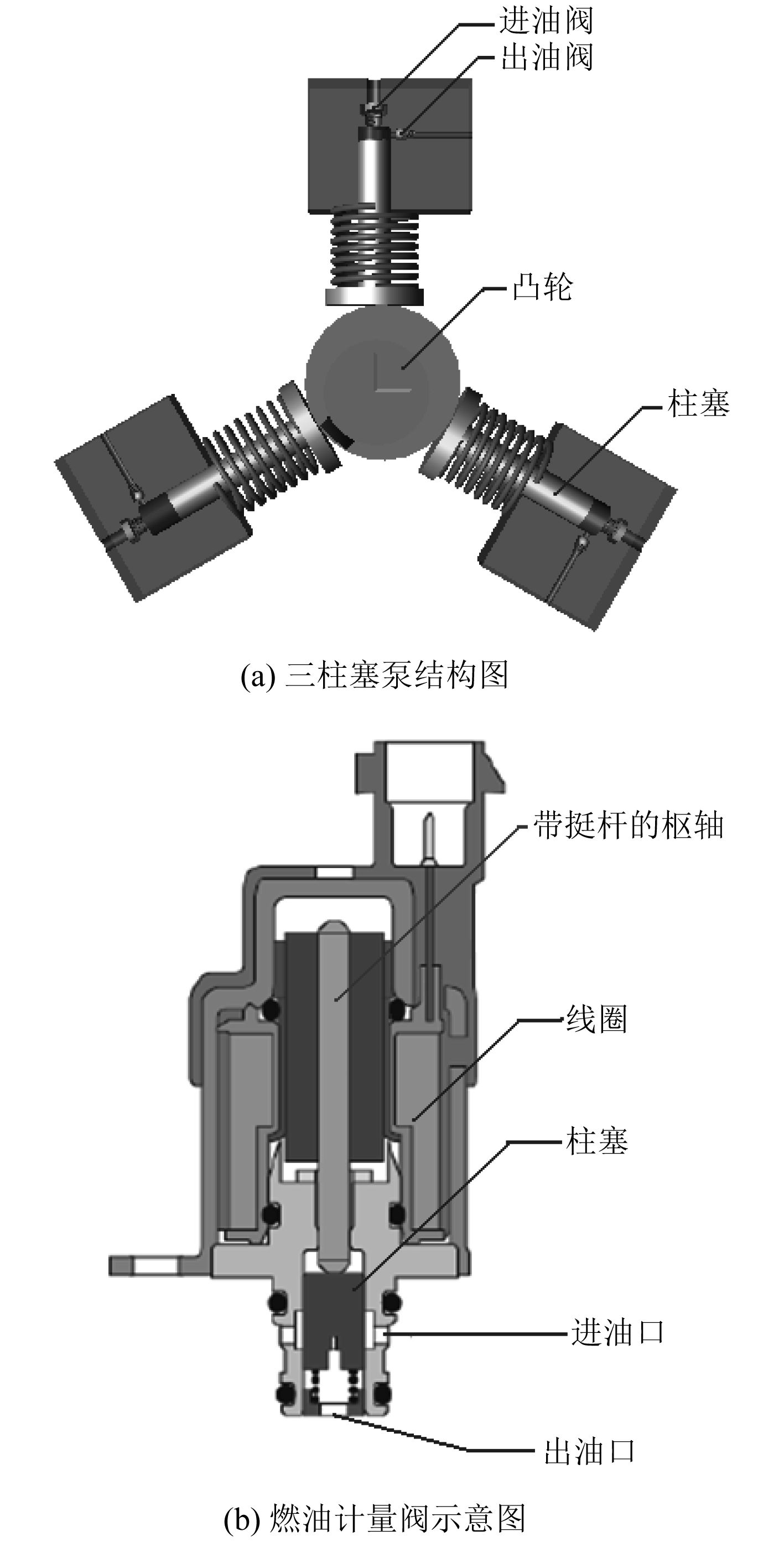图 1 高压油泵结构示意图 Fig. 1 Structure schematic diagram of high pressure fuel pump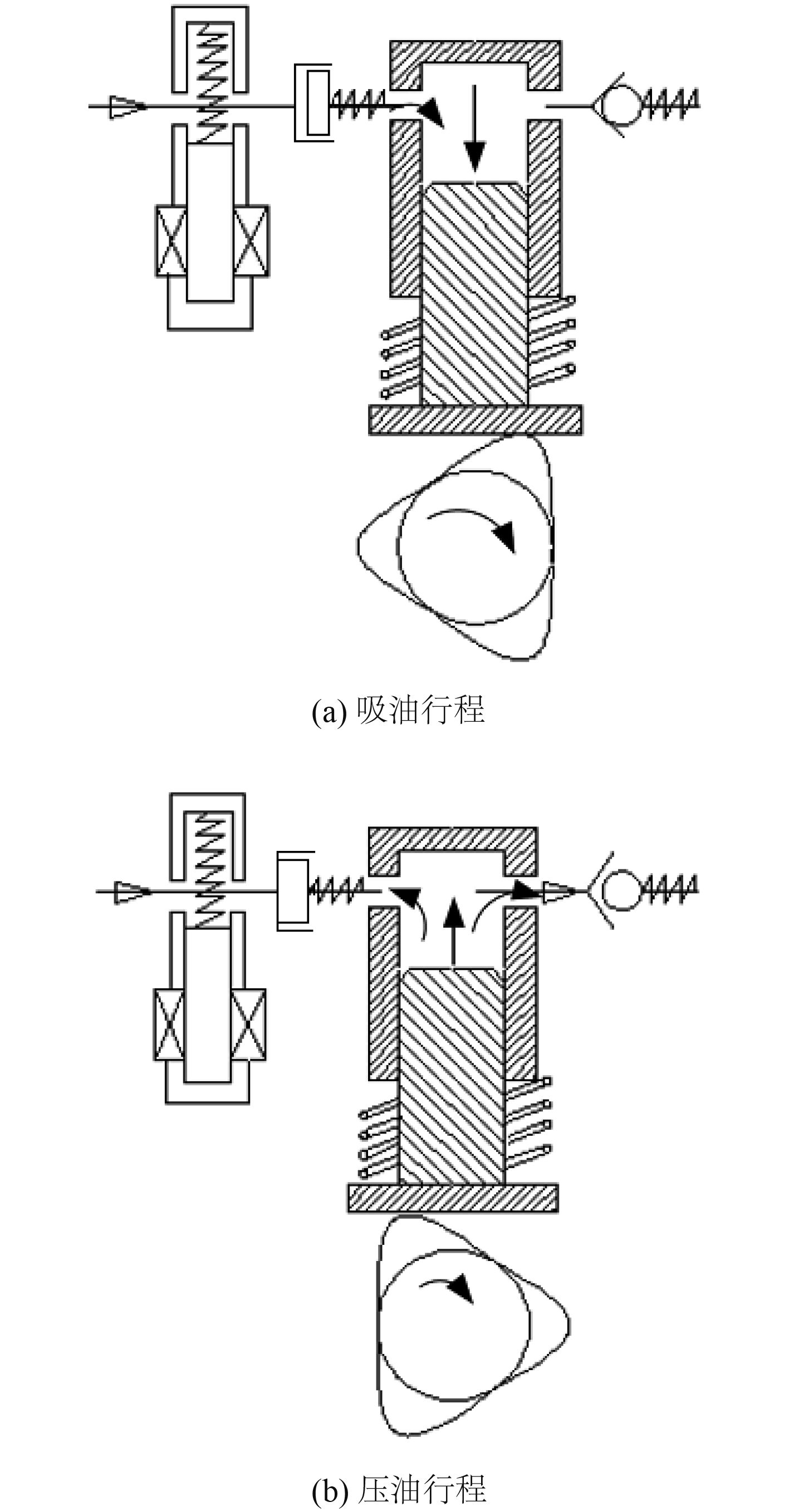图 2 高压油泵工作原理示意图 Fig. 2 Schematic diagram of high pressure pump working principle
2 仿真模型建立及验证 2.1 模型建立

 ${Q_H} = {Q_{VH}} + {Q_{HR}} + {Q_{LH}} + {Q_{HO}},$ (1)

 ${Q_H} = {A_H}\frac{{{\rm d}{H_P}}}{{{\rm d}t}},$ (2)

 ${Q_{VH}} = \frac{{{V_H}}}{E}\frac{{{\rm d}{P_H}}}{{{\rm d}t}},$ (3)

 ${Q_{HR}} = \xi \left( {\mu {A_{HR}}} \right)\sqrt {\frac{2}{\rho }\left| {{P_H} - {P_{\rm{R}}}} \right|},$ (4)

 ${Q_{LH}} = \gamma \left( {\mu {A_{LH}}} \right)\sqrt {\frac{2}{\rho }\left| {{P_H} - {P_L}} \right|} ,$ (5)

 ${Q_{HO}} = \frac{{π {d_H}\delta _H^3}}{{12\eta {L_H}}}\left( {{P_H} - {P_0}} \right),$ (6)

 ${U_c} = Ri + N\frac{{{\rm d}\varphi }}{{{\rm d}t}},$ (7)
 ${F_{mag}} = \frac{{{\mu _0}{{(iN)}^2}{S_a}}}{{2{{(\delta - x)}^2}}},$ (8)
 ${F_h} = A{P_{con}},$ (9)
 ${F_{mag}} - {F_{pre}} - kx + {F_h} - {\lambda _x}\frac{{{\rm d}x}}{{{\rm d}t}} = m\frac{{{{\rm d}^2}x}}{{{\rm d}{t^2}}}{\text{。}}$ (10)

 $\frac{{{V_R}}}{E}\frac{{{\rm d}{P_R}}}{{{\rm d}t}} = {Q_{HR}} - {Q_{OUT}}{\text{。}}$ (11)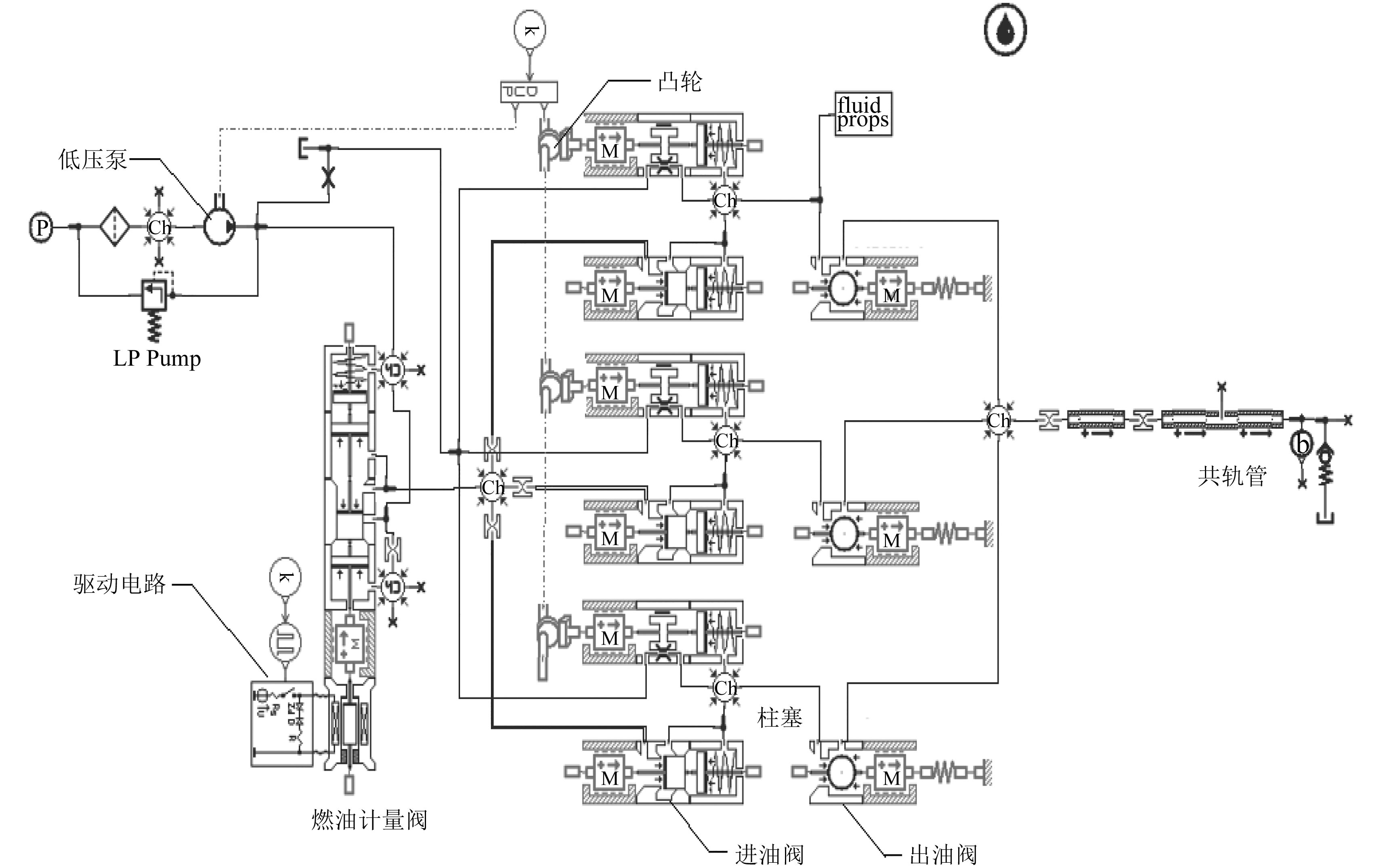图 3 高压油泵及共轨管的仿真模型 Fig. 3 Simulation model of high pressure fuel pump and common rail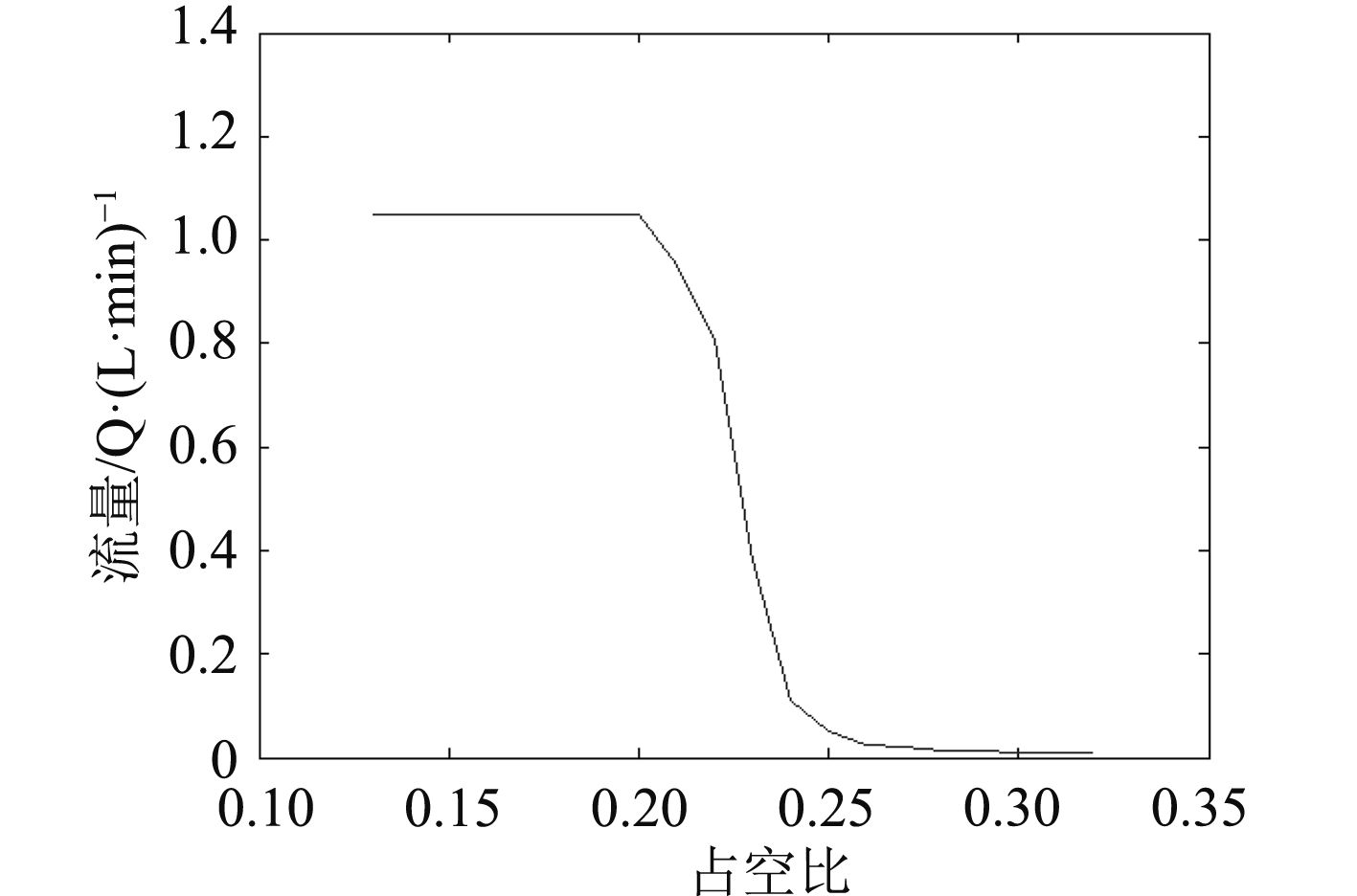图 4 高压油泵的流量特性曲线 Fig. 4 Flow chrarcteristic curve of high pressure fuel pump
2.2 模型验证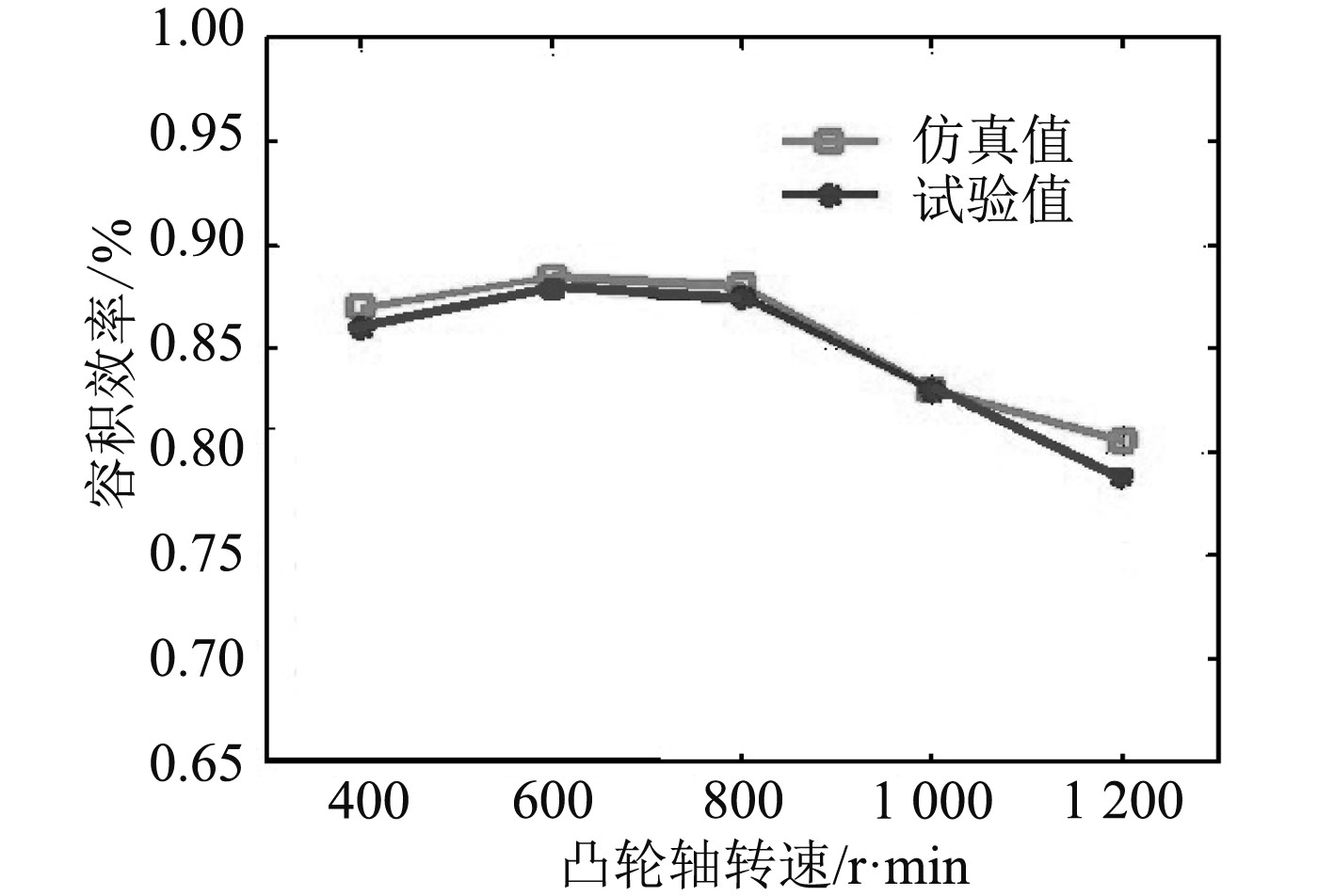图 5 容积效率试验值与仿真值对比图 Fig. 5 Volumetric efficiency comparison between simulation results and experimental results
3 高压油泵动态特性分析

3.1 控制信号频率和驱动轴转速不变，控制信号占空比变化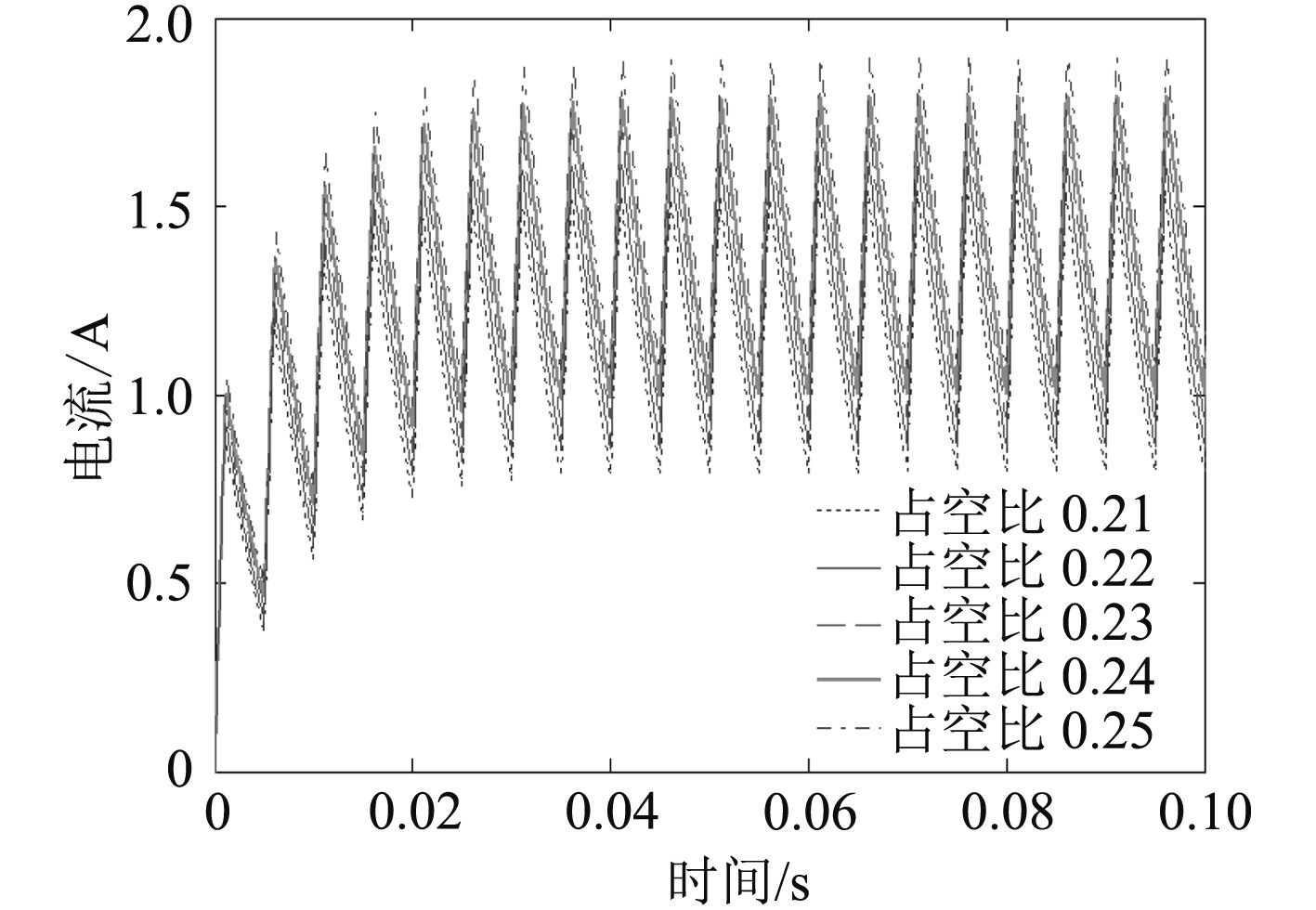图 6 控制信号占空比对电流的影响 Fig. 6 Influence of control signal duty ratio on current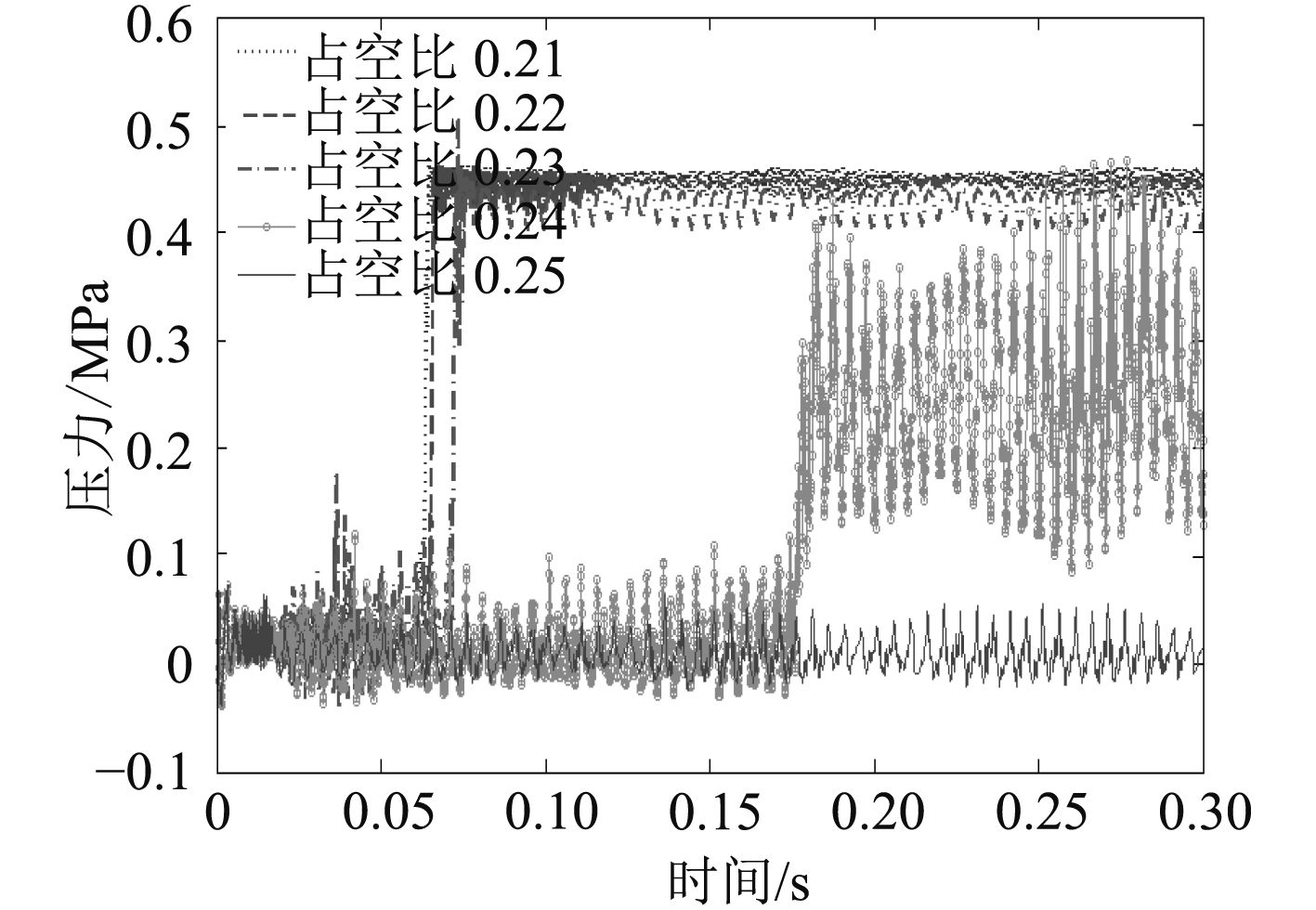图 7 控制信号占空比对输入燃油压力的影响 Fig. 7 Effect of control signal duty ratio on input fuel pressure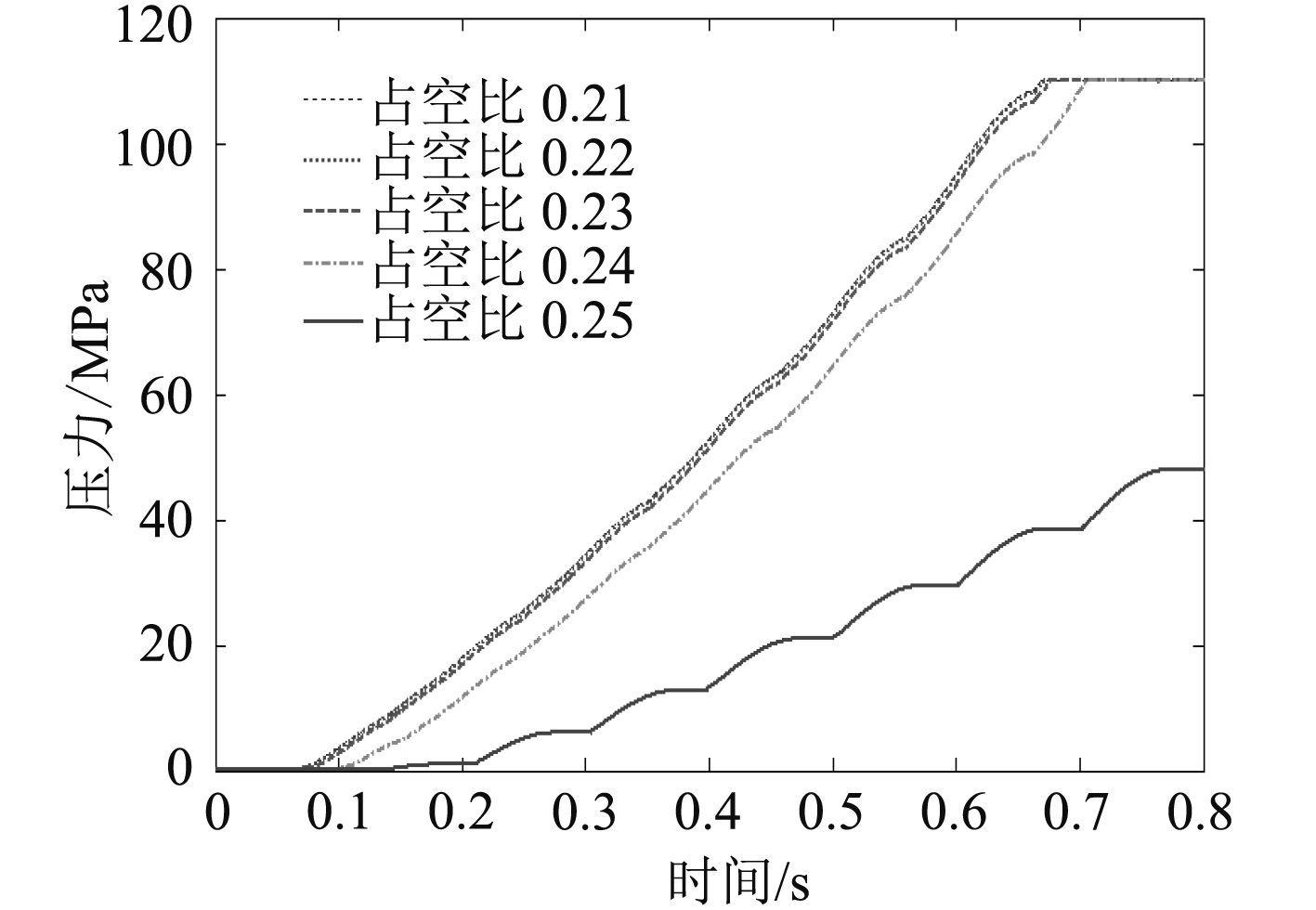图 8 控制信号占空比对输出燃油压力的影响 Fig. 8 Effect of control signal duty ratio on output fuel pressure

3.2 控制信号占空比和驱动轴转速不变，控制信号频率变化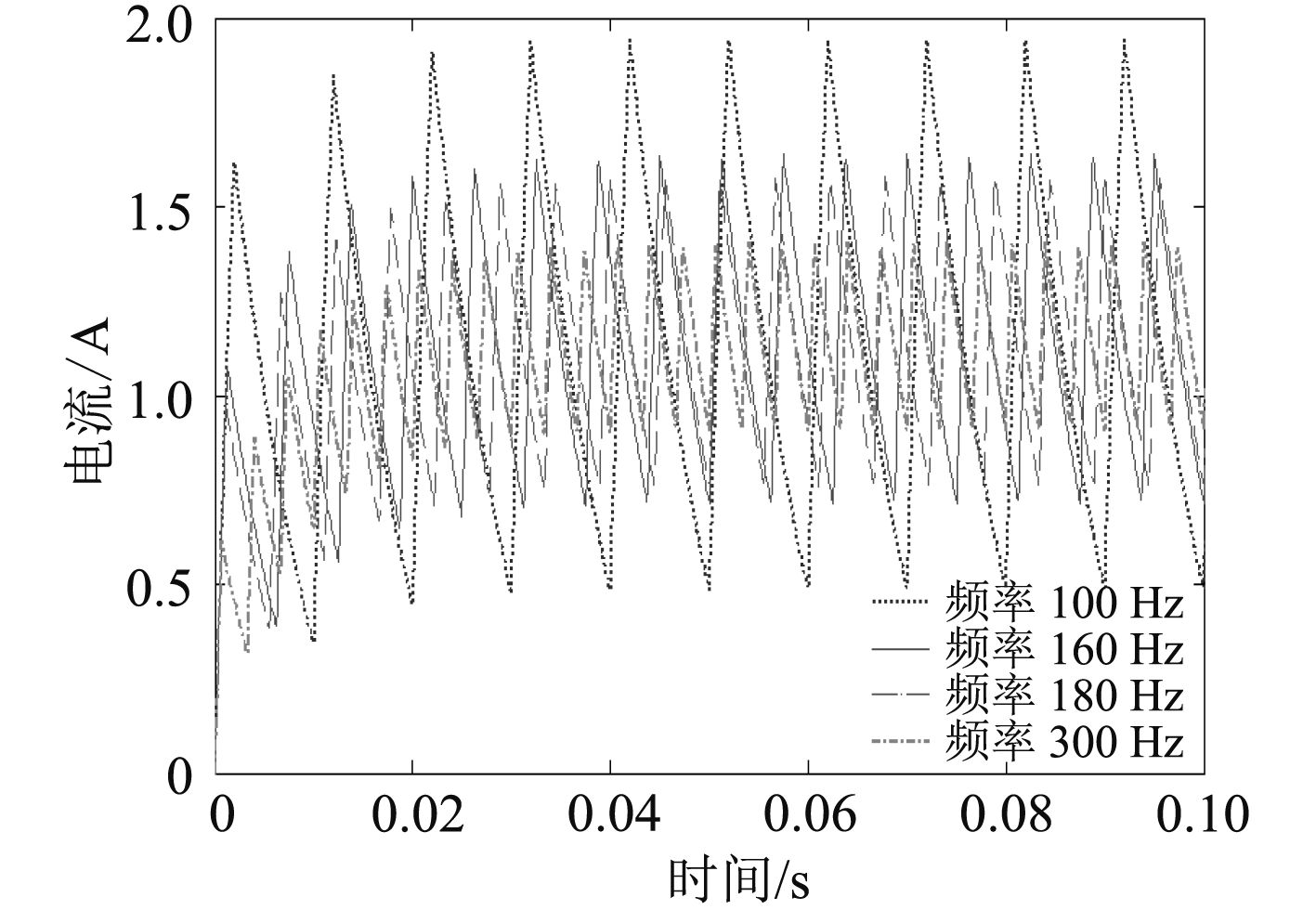图 9 控制信号频率对电流的影响 Fig. 9 Influence of control signal frequency on current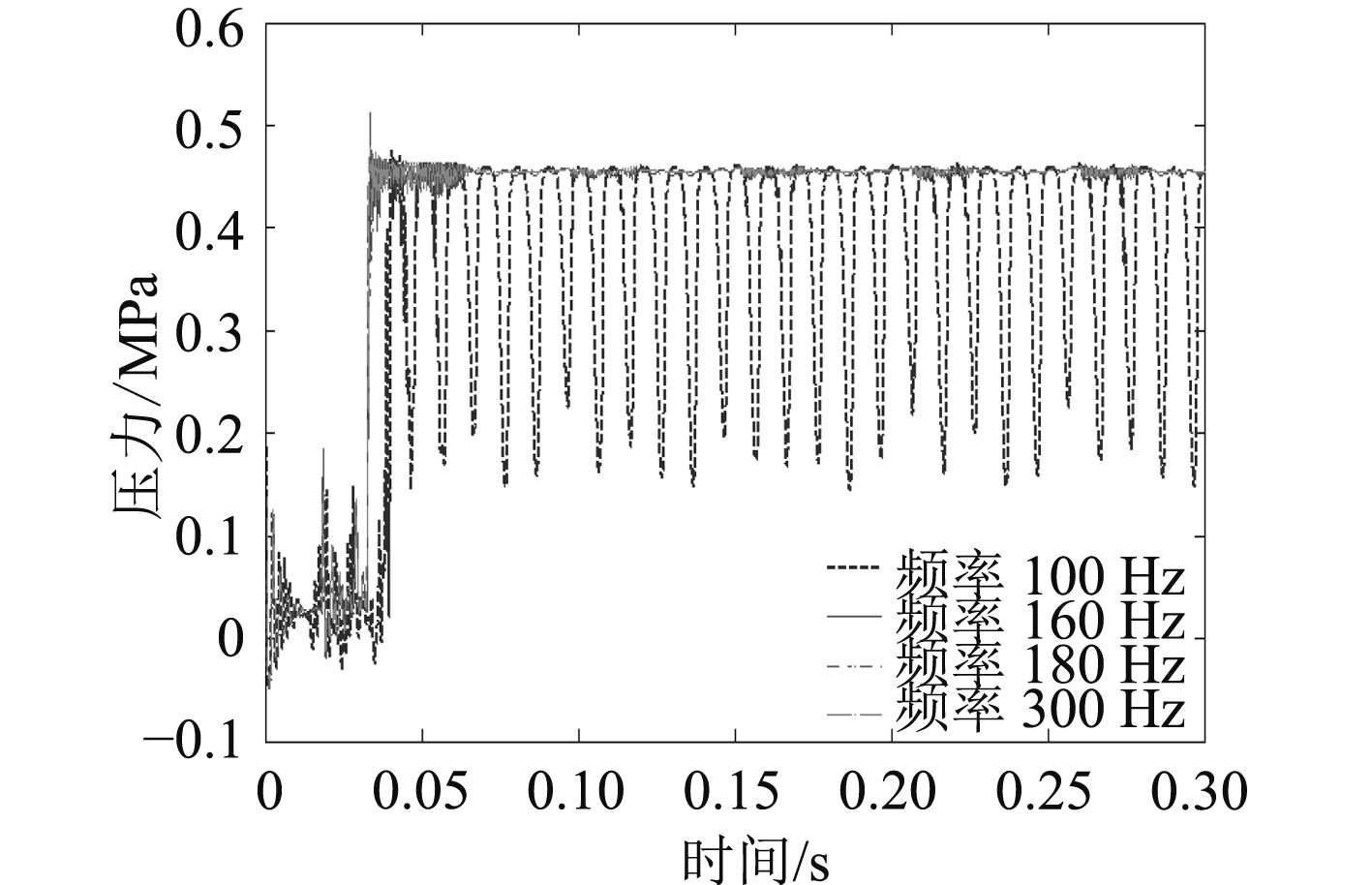图 10 控制信号频率对输入燃油压力的影响 Fig. 10 Effect of control signal frequency on input fuel pressure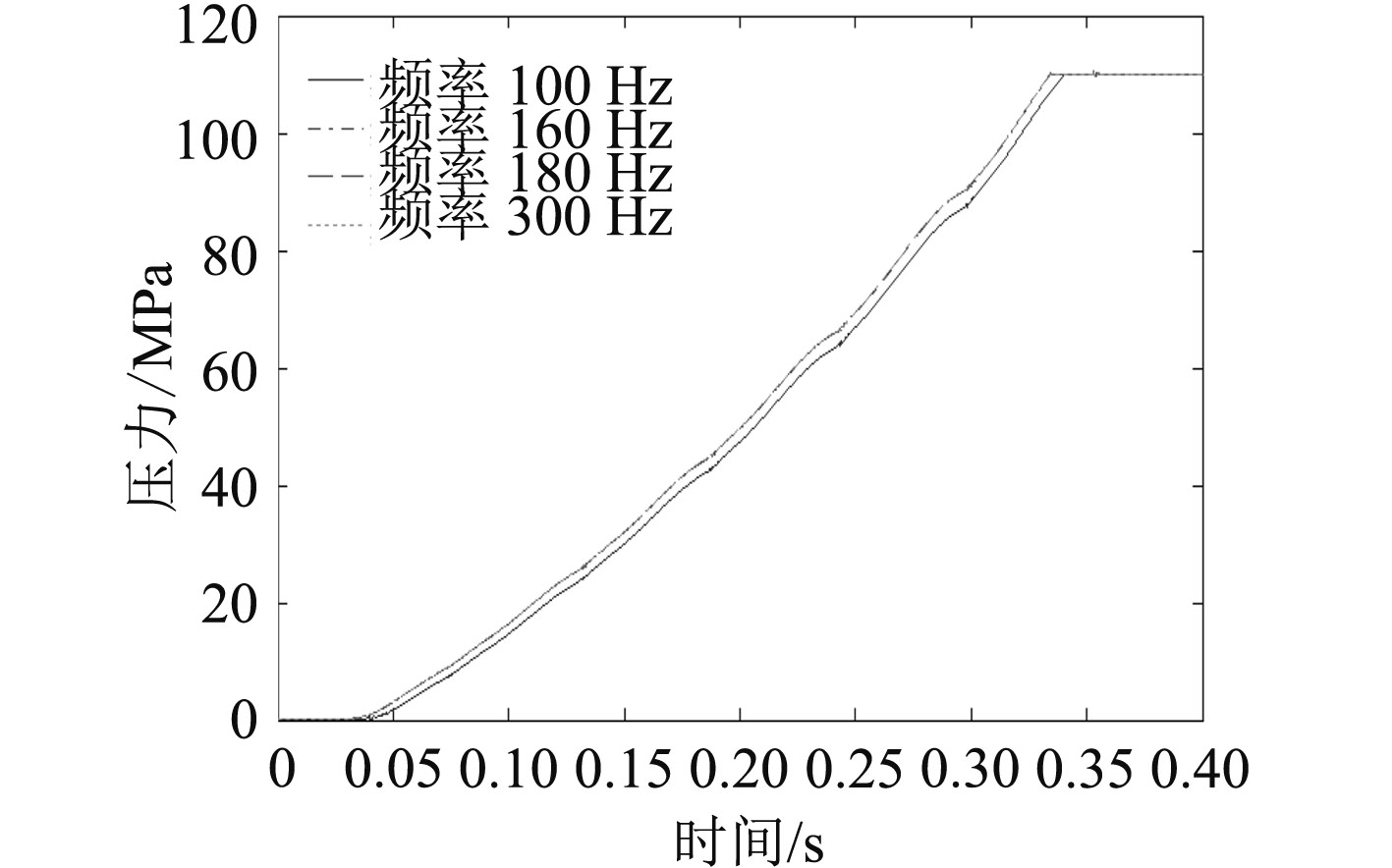图 11 控制信号频率对输出燃油压力的影响 Fig. 11 Effect of control signal frequency on output fuel pressure

3.3 控制信号不变，驱动轴转速变化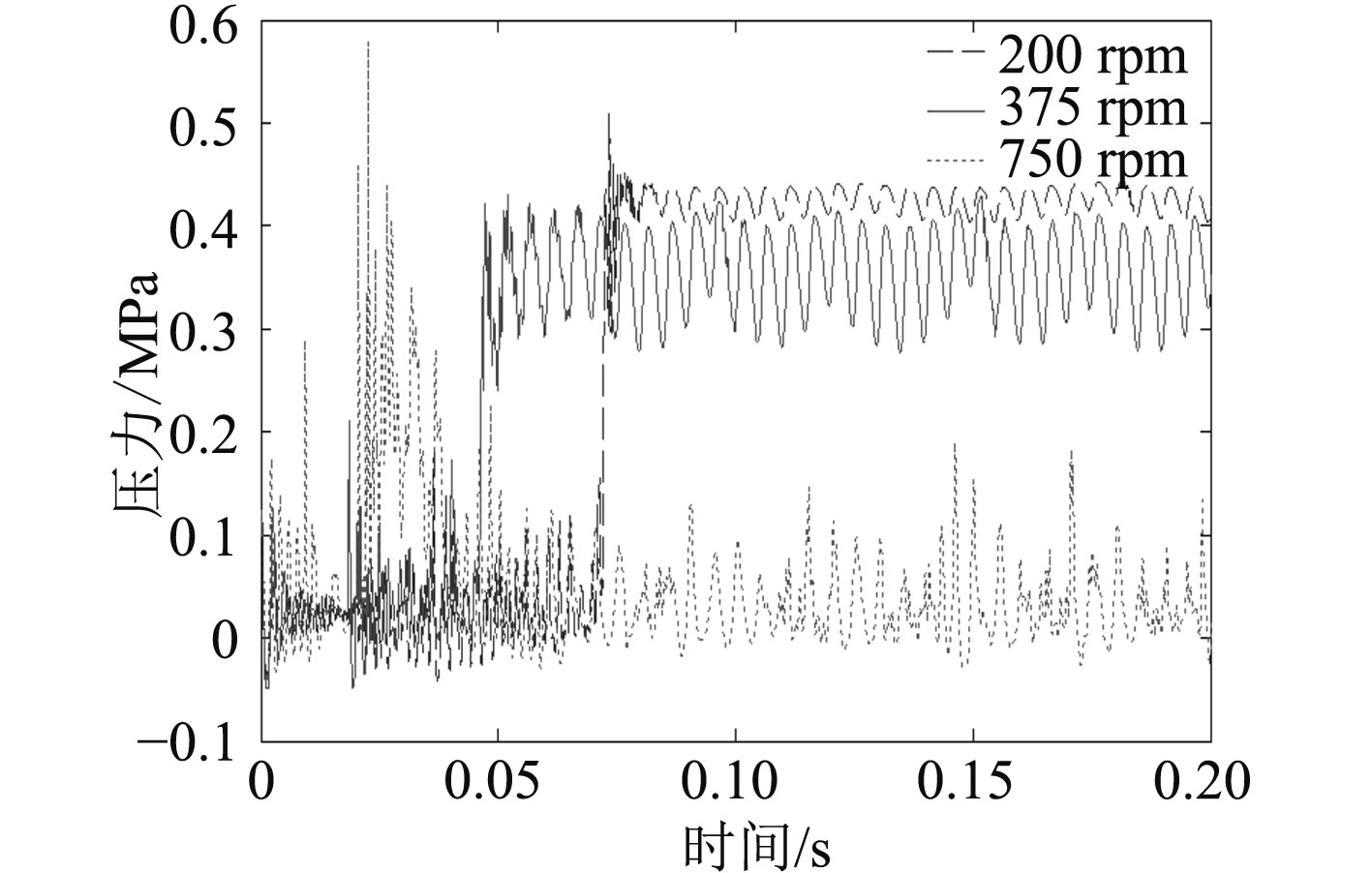图 12 驱动轴转速对输入燃油压力的影响 Fig. 12 Effect of drive shaft speed on input fuel pressure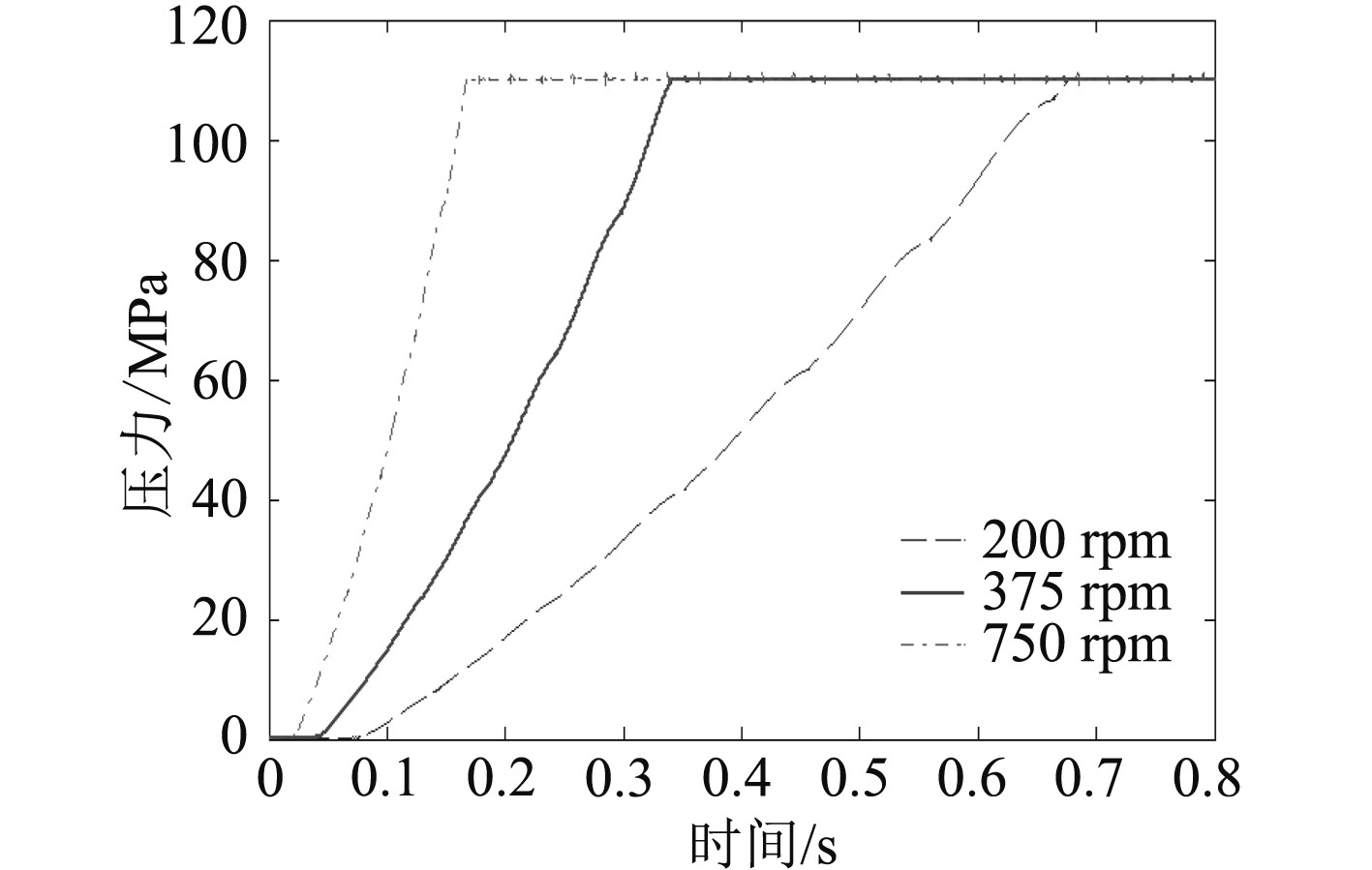图 13 驱动轴转速对输出燃油压力的影响 Fig. 13 Effect of drive shaft speed on output fuel pressure

4 结 语

1）在介绍基于燃油计量阀的高压油泵工作原理的基础上，利用AMESim软件建立了高压油泵及共轨管的仿真模型，与在高压油泵性能试验中得到的容积效率对比，表明该模型可准确预测高压油泵的动态特性。

2）燃油计量阀控制信号的频率对高压油泵动态特性的影响较小，而燃油计量阀控制信号的占空比和驱动轴转速对高压油泵动态特性的影响较大。随着燃油计量阀控制信号占空比的减小以及驱动轴转速的增大，高压油泵输出高压燃油的响应加快，泵油能力增强。

3）该研究为基于燃油计量阀的高压油泵初步设计和选型提供了理论依据，同时为高压共轨喷油系统的快速启动以及共轨管内压力的稳定控制奠定基础。

  PARK S H, KIM H J, LEE C S. Comparison of experimental and predicted atomization characteristics of high-pressure diesel spray under various fuel and ambient temperature[J]. Machinery, Science and Technology, 2010, 24 (7): 1491–1499. DOI: 10.1007/s12206-010-0417-1  陈永贤, 于文斌, 郭树满, 等. 若干燃烧控制参数对柴油机低负荷排放特性和效率影响的试验[J]. 内燃机学报, 2011, 29 (3): 193–199.  JOONSIK H, DONGHUI Q, YONGJIN J, et al. Effect of injection parameters on the combustion and emission characteristics in a common-rail direct injection diesel engine fueled with waste cooking fuel biodiesel[J]. Renewable Energy, 2014, 63 : 9–17. DOI: 10.1016/j.renene.2013.08.051  欧阳光耀, 安士杰, 刘振明, 等. 柴油机高压共轨喷射技术[M]. 北京: 国防工业出版社, 2012.  TENG H, MCCANDLESS J. performance analysis of rail-pressure supply pumps of common-rail fuel systems for diesel engines[C]// SAE paper, petroit, michigan, USA, 2005–01-0909, 2005.  范立云, 连历, 马修真, 等. 高压共轨系统高压油泵供油特性影响因素分析[J]. 内燃机工程, 2016, 37 (4): 219–226.  YAN J W. Common rail injection system iterative learning control based parameter calibration for accurate fuel injection quantity control[J]. International Journal of Automotive Technology, 2011, 12 (2): 149–157. DOI: 10.1007/s12239-011-0019-7  倪成群, 崔国旭, 张雁桥, 等. 柴油机高压共轨系统高压油泵驱动扭矩仿真研究[J]. 车用发动机, 2010 (3): 188–191.  KENDLBACHER C, LENGENFELDER T, BLATTERER D, et al. The 2200 bar modular common rail injection system for large diesel and HFO engines[C]// 27th CIMAC Congress, Shanghai, 2013.  李鸿怀. 柴油机高压共轨系统多工况轨压控制策略研究[D]. 无锡: 江南大学, 2011.  NDIAYE E, BAZILE J P, NASRI D, et al. High pressure thermophysical characterization of fuel used for testing and calibrating diesel injection systems[J]. Fuel, 2012, 98 : 288–294. DOI: 10.1016/j.fuel.2012.04.005